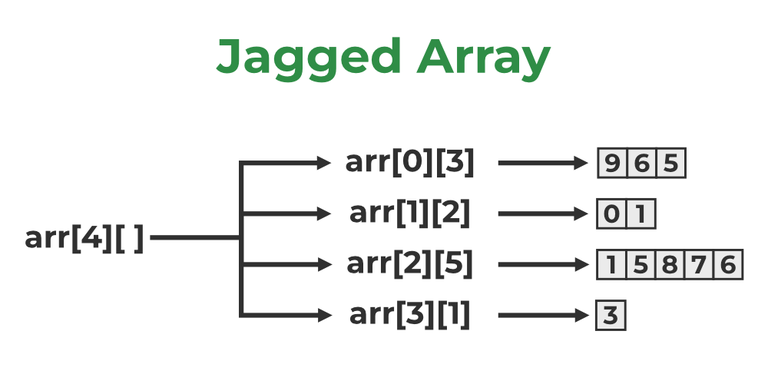GeeksforGeeks App
Open AppBrowser
Continue

Prerequisite:

## What is a Jagged Array?

A jagged array is an array of arrays such that member arrays can be of different sizes, in 2D array terms for each row we can have a variable number of columns. These types of arrays are also known as Jagged arrays.Jagged Array in C++

Example:

```arr[] = 1 2 3 4        // arr : 1st row have 4 columns
5 6            // arr : 2nd row have 2 columns
7 8 9          // arr : 3rd row have 3 columns```

## Methods to Create Jagged Array

Jagged Array can be implemented in C++ in two ways:

1. Using a static array of pointers
2. Using dynamic 2D arrays

### 1. Using a static array of pointers

• create ‘n’ numbers 1D-arrays (row1, row2, row3, ….. etc.) where n is no of rows, the size of each row array will be No. of columns i.e, the number of elements in each row array will show no. of columns in that particular row.
• Create a 1D array of pointers, storing the base address of each row array.
• Create another 1D array Sizes[] storing the size of each row array (This helps while iterating each element).

To know about the array of pointers refer to an array of pointers article.

Below is the implementation of the above method:

## C++

 `// C++ Program to implement  Jagged Array``// 1st way: static arrays``#include ``using` `namespace` `std;`` ` `int` `main()``{``    ``// create 3 row arrays having different sizes``    ``// ( no ofcolumns)``    ``int` `row1[] = { 1, 2, 3, 4 };``    ``int` `row2[] = { 5, 6 };``    ``int` `row3[] = { 7, 8, 9 };`` ` `    ``// storing base address of each row array``    ``int``* jagged[] = { row1, row2, row3 };`` ` `    ``int` `sizes[] = { 4, 2, 3 };`` ` `    ``cout << ``"elements in matrix form as follow"` `<< endl;``    ``for` `(``int` `i = 0; i < 3; i++) {`` ` `        ``// getting current(ith) row``        ``int``* ptr = jagged[i];`` ` `        ``for` `(``int` `j = 0; j < sizes[i]; j++) {``            ``// for ith row having sizes[i] no. of``            ``// columns`` ` `            ``cout << *(ptr + j) << ``" "``;``            ``// *ptr have base address``            ``// adding j means access jth``            ``// element for particular(ith) row``        ``}``        ``cout << endl;``    ``}`` ` `    ``return` `0;``}`

Output

```elements in matrix form as follow
1 2 3 4
5 6
7 8 9 ```

### 2. Using Dynamic 2D arrays

• Create row, col variables storing no. of rows and no. of columns.
• Create a Dynamic row array arr (array of pointers) that can store a “row” number of addresses.
• Store size in another 1D array Sizes[] (size of no. or Rows) to store no. of columns for each row element in the row array. (This helps while iterating each column for each row).
• Create a Dynamic Column array for each row element with size: sizes[i]
• Now each row element in the Row array has the Base address of each Column array.

Below is the implementation of the above method:

## C++

 `// C++ Program to implement Jagged array``// 2nd way: Dynamic 2D array``#include ``using` `namespace` `std;`` ` `int` `main()``{``    ``// system("cls");`` ` `    ``int` `row, col;``    ``row = 3;`` ` `    ``// Create Row Array- dynamic array of pointers``    ``int``** arr = ``new` `int``*[row];``    ``int` `sizes[] = { 4, 2, 3 };``    ``// int *sizes= new int[row];``    ``// if taking row as input`` ` `    ``// no of columns for each row as input from user``    ``for` `(``int` `i = 0; i < row; i++) {``        ``/*``        ``cin>>col;        //if col is taken as input``        ``/ sizes[i]=col;``        ``// store each col number in size ( if row and col``        ``// are taken as input)``        ``*/`` ` `        ``*(arr + i) = ``new` `int``[sizes[i]];``        ``// creating column of sizes[i] for each row``    ``}`` ` `    ``// input from user``    ``int` `num = 1;``    ``for` `(``int` `i = 0; i < row; i++) {``        ``for` `(``int` `j = 0; j < sizes[i]; j++) {``            ``// cin>>arr[i][j];    //if user want to input``            ``arr[i][j] = num++;``        ``}``    ``}`` ` `    ``cout << ``"elements in matrix form as follow"` `<< endl;``    ``for` `(``int` `i = 0; i < row; i++) {``        ``for` `(``int` `j = 0; j < sizes[i]; j++) {``            ``cout << arr[i][j] << ``" "``;``        ``}``        ``cout << endl;``    ``}`` ` `    ``return` `0;``}`

Output

```elements in matrix form as follow
1 2 3 4
5 6
7 8 9 ```

My Personal Notes arrow_drop_up